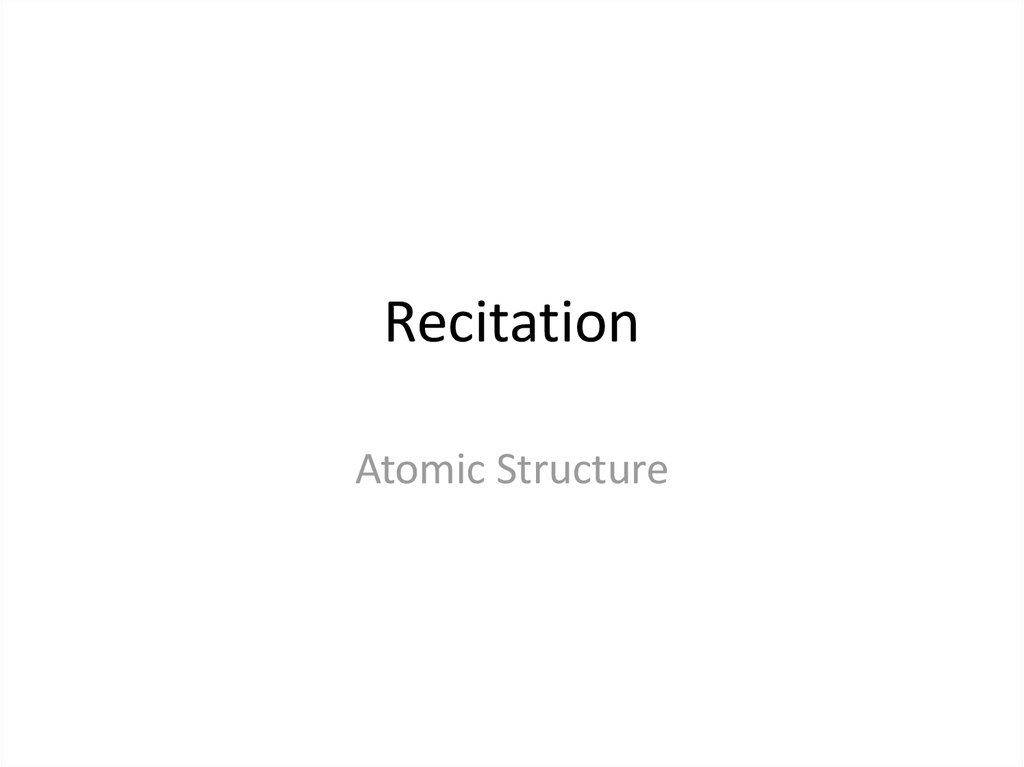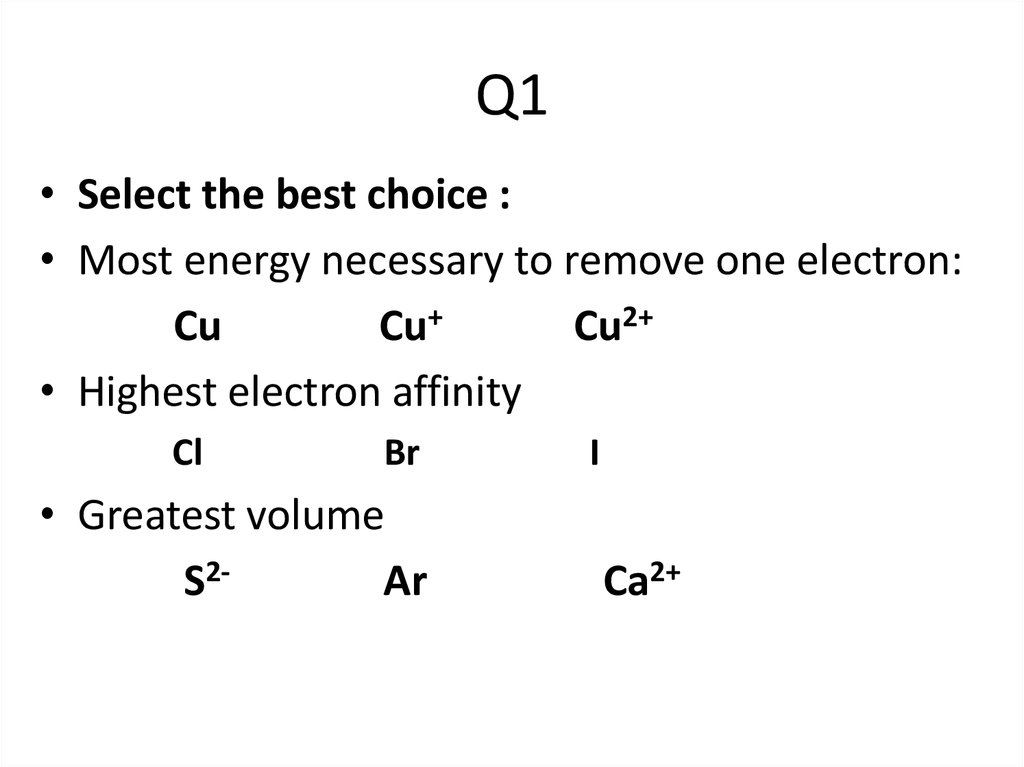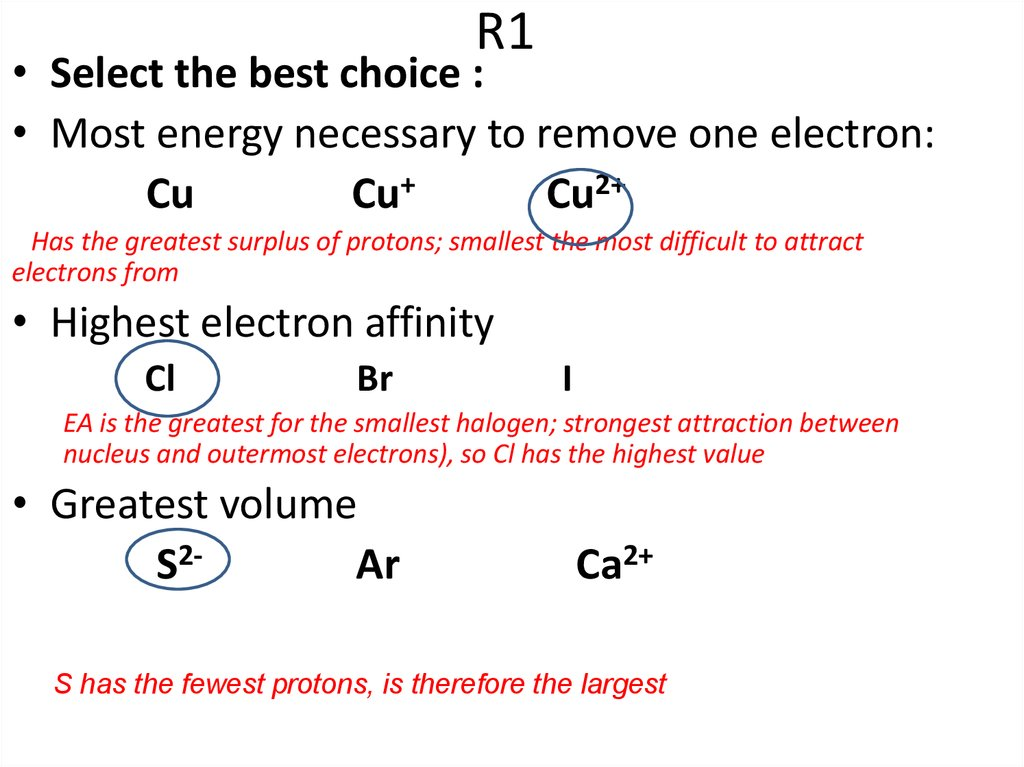# Atomic structure

Atomic Structure

## 2. Q1

• Select the best choice :
• Most energy necessary to remove one electron:
Cu
Cu+
Cu2+
• Highest electron affinity
Cl
Br
• Greatest volume
S2Ar
I
Ca2+

## 3. R1

• Select the best choice :
• Most energy necessary to remove one electron:
Cu
Cu+
Cu2+
Has the greatest surplus of protons; smallest the most difficult to attract
electrons from
• Highest electron affinity
Cl
Br
I
EA is the greatest for the smallest halogen; strongest attraction between
nucleus and outermost electrons), so Cl has the highest value
• Greatest volume
S2Ar
Ca2+
S has the fewest protons, is therefore the largest

## 4. Q2

• Explain why Ag+ is the most common ion for silver.
• Which is the more likely configuration for Mn2+ :
[Ar]4S23d3 or [Ar]3d5 .

## 5. R2

• Ag+ is the most common ion for silver because it
has [Kr]4d10 . With filled 4d subshells
(0.25pts)

## 6. R1C

• The preferred configuration
of Mn2+ is [Ar]3d5
The 3d orbital are lower in energy than the 4s. In
addition, the configuration minimizes electronelectron repulsions (because each d electron is
in a separate space) and maximizes the
stabilizing effect of electrons with parallel spin
e)

## 7. 3.2 Units

A) Electromagnetic Radiation
3.2.1: Electromagnetic Radiation
3.2.2: Quantization
3.2.3: The Atomic Spectrum of Hydrogen

## 8. Spectrum

Röntgen J. W. Ritter
Rutherford
1995
1801,
W. Herschel
1800,
Hertz in 1887
1862, Maxwell (visible and invisible are EM radiation)
8

9

## 10. 3.2 EM Radiation

A) Electromagnetic Radiation
• The frequency of radiation used in a typical microwave
oven is 1.00 1011 Hz. What is the energy of a mole of
microwave photons with this frequency
a. 39.9 J mol–1
b. 3.99 J mol–1
c. 399 J mol–1
d. 0.39 J mol–1

## 11. 3.2 Units

A) Electromagnetic Radiation
• The frequency of radiation used in a typical microwave
oven is 1.00 1011 Hz. What is the energy of a mole of
microwave photons with this frequency
h, the Planck constant = 6.626 10–34 J s
Avogadro constant, 6.022 1023 mol–1

## 12. Solution 1

E = h ,
Multiply this value by the Avogadro constant to find the
energy of a mole of photons (where the Avogadro
constant, NA, is the number of entities in a mole.
E = h , to calculate the energy of one photon where
• h, the Planck constant = 6.626 10–34 J s
• = 1.00 1011 Hz (s–1)
• E = (6.626 10–34 J s) (1.00 1011 s–1) E =
6.63 10–23 J
• The value for a single photon can then be converted into
the energy for one mole of photons by multiplying by
the Avogadro constant, 6.022 1023 mol–1

a. 39.9 J mol–1
b. 3.99 J mol–1
c. 399 J mol–1
d. 0.39 J mol–1

## 14. 3.2 Atomic Spectra

A) What is the ionization energy (kJ/mol) for an
excited state of hydrogen in which the electron
has already been promoted to n = 2 level?
RH = 3.29 x 1015 Hz
(Hz = s-1)
h = 6.626 x 10-34 Js
Avogadro Constant = 6.022 x 1023 mol-1
E NAh

A
3.2 Response

A
3.2 Response
E NAh

## 17. Exercise

A line from the Pfund series has the frequency 8.02
1013 Hz. What value of n2 generates this line in
the spectrum?
a. 5
b. 6
c. 7
d. 8

18

## 19. Exercise

A line from the Pfund series has the frequency 8.02
1013 Hz. What value of n2 generates this line in
the spectrum?
1
1
2 2
RH n1 n2
1
8.02 1013 Hz
1
2 2
15
3.29 10 Hz
n2
5
1
1
1
0.02438 2 0.04 2
n2
25 n2

## 20. Exercise

A line from the Pfund series has the frequency 8.02
1013 Hz. What value of n2 generates this line in
the spectrum?
1
1
2 2
RH n1 n2
1
8.02 1013 Hz
1
2 2
15
3.29 10 Hz
n2
5
1
1
1
0.02438 2 0.04 2
n2
25 n2

## 21. Exercise

Rearranging, by taking 0.04 from each side, gives
1
0.01563 2
n2
Dividing both sides by –0.01563 and multiplying by n2 gives
a. 5
b. 6
c. 7
d. 8
n2
2
1
64
0.01563
n2 = 8

## 22. 3.2 Light Interference

In Thomas Young’s experiment when he passed
light through two closely placed slits, it gave an
interference pattern. This evidence suggests
that light behaves as a particle.
True
false?

## 23. 1.2 Light Interference

false?
A set of maxima and minima in an interference
pattern suggests a totally different effect. As shown
in Figures 3.7 and 3.8 (p.114), this experiment is
demonstrating the wave like properties of light,
where the interference pattern is generated from
individual waves adding together (in phase) or
subtracting from one another (out of phase).

## 24. Q4

The wave function of an electron is related to the probability for
finding a particle in a given region of space.
The relationship is given by:
If we integrate the square of the wave function over a given
volume we find the probability that the particle is in that volume.
In order for this to be true the integral over all space must be
one.
The volume element can be given by
Find X of the integration above. Show your integration steps

## 25.

Radial Probability Distribution
The product of the three sides of the cube is
equivalent to